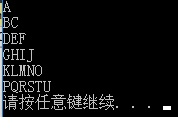A
BC
DEF
GHIJ
KLMNO
PQRSTU

#include
int main(void)
{
int i,j;
char a = 'A';

``````for (i = 0; i < 6; i++,a++) {
for (j = 0; j < i + 1; j++)
printf("%c", a + j);
printf("\n");
}
getchar();
return 0;
``````

3个回答

``````
#include "stdafx.h"
#define N 6

int main()
{
char a = 'A';

for (int i = 0; i < N; i++) {
for (int j = 0; j <= i; j++) {
printf("%c", a++);
}
printf("\n");
}
return 0;
}
```````````` #include <stdio.h>
#include <math.h>
int main()
{
char c,c1='A';int n=0;
for (c = 'A'; c <= 'U'; c++)
{
printf("%c", c);
if (c-c1==n) { printf("\n"); n++; c1=c+1;}
}
}

``````

http://ideone.com/Nh6ZmL
A
BC
DEF
GHIJ
KLMNO
PQRSTU

#include "stdafx.h"
#define N 6

int main()
{
char a = 'A';

``````for (int i = 0; i < N; i++) {
for (int j = 0; j <= i; j++) {
printf("%c", a++);
}
printf("\n");
}
return 0;
``````

}